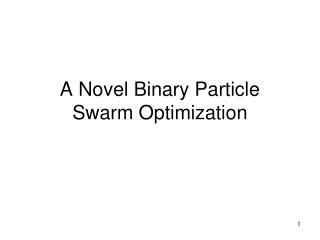DownloadDownload PresentationA Novel Binary Particle Swarm Optimization

# A Novel Binary Particle Swarm Optimization

Download Presentation## A Novel Binary Particle Swarm Optimization

- - - - - - - - - - - - - - - - - - - - - - - - - - - E N D - - - - - - - - - - - - - - - - - - - - - - - - - - -
##### Presentation Transcript

1. A Novel Binary Particle Swarm Optimization

2. Binary PSO- One version • In this version of PSO, each solution in the population is a binary string. • Each binary string is of dimension n which is evaluated to give parameter values. • In the binary PSO, each binary string represents a particle • Strings are updated bit-by-bit based on its current value, the value of that bit in the best (fitness) of that particle to date, and the best value of that bit to date of its neighbors

3. Binary PSO- What is a neighbor? • For binary strings, neighbors can be selected in one of several ways. Some examples are: (for a neighborhood of size k) • Neighbors are the k binary strings whose Hamming distance is minimum • For equal Hamming distances, the choices are arbitrary • In the beginning, arbitrarily assign groups of k strings to neighborhoods • Let the neighborhood size be the population size

4. BPSO • In regular (real valued) PSO, everything is in terms of a velocity. • In BPSO, how does one define a velocity for a single bit? • Generally the velocity is defined in terms of a probability of the bit changing • You will see in a minute how this works

5. BPSO • As just noted, in BPSO, bit-by-bit updates are done probabilistically • In other words, for a chosen bit (d) in a chosen string (i) it is changed to a 1 with a probability (P) that is a function of its predisposition to be a 1, the best value of itself to date, and the best value of its neighbors. • 1-P is the probability of changing to a 0 • Once P is determined, we generate a random number R, and if R<P, then the bit becomes a 1; otherwise it becomes a 0

6. BPSO • The formula for an individual bit’s update is: • The function P is a probability, and thus once this value is computed for a given particle bit, we must generate a uniform random number to see whether it should be a 1 or a 0

7. BPSO

8. BPSO • The challenge is to come up with the f() from the previous slide • The value of vid(t) determines a string’s propensity to choose 1 or 0. • Higher values of vid(t) mean it is more likely to choose a 1, similarly for lower values choosing a 0

9. BPSO • For the function • We are saying that this probability is a function of the bit’s current value, its “velocity” and the values of the best to date for the bit and best to date for the neighborhood. • Remember, best to date for a bit is simply a 0 or a 1

10. BPSO • Since f will be a probability value, we know it must range between 0 and 1. • There are several measures or expressions used for f, one that is commonly used is the sigmoid function

11. BPSO • In the preceding • Sometimes these parameters are chosen from a uniform distribution 0 - 2, such that the sum of their two limits is 4.0

12. BPSO Example • As an example, let’s say that we are dealing with a population of 5 bit binary particles and a population of 4 particles 10101 01011 11100 01101 • We are updating particle 2 (01011), bit 3 (0)

13. BPSO Example • Furthermore, we will assume that the current propensity (velocity) of this bit to be a 1 is 0.25. • Furthermore, assume that the best value of this particle (to date) was 00100 • And the best value of the whole population (to date) was 01111

14. BPSO Example • Thus we have:

15. BPSO Example • Now, with the value for f, we generate a random number, and if it is < f then bit x becomes a 1 otherwise, it becomes a 0.

16. BPSO - Parameters • Sometimes the v value is limited so that f does not approach too closely to 0.0 or 1.0. • In this case, constant parameters [Vmin ,Vmax] is used. • When vid is > Vmax, vid is set to Vmax • When vid is < Vmin, vid is set to Vmin

17. BPSO - Initializing • There are a few things that need to be initialized. • Initial population (particle) values – just randomly generate binary strings • Initial velocities can be generated as

18. Main problems with binary PSO • Parameters of the binary PSO • the effects of these parameters are the opposite of those for the real valued PSO • values ofw < 1 prevents convergence. For values of −1<w <1,Vij becomes 0 over time. for w < 1 we have • Memory of BPSO

19. Proposed Binary Particle Swarm Optimization • Two velocities for PSO

20. Results